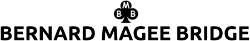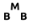Week 0144 : 27th March 2023

Search

# LOSING TRICK COUNT

A method of evaluating the playing strength of a hand, for trump contracts, based on the number of expected losers.

You count the number of losers as follows: With three or more cards, the number of losers in a suit is normally equal to the number of missing high honours (the ace, king and queen) eg A-x-x counts as two losers, K-Q-x or K-Q-x-x count as one loser.

With a doubleton, the queen normally counts as a small card in the above calculation, and similarly with any singleton, other than the ace, the suit counts as one loser.

Exceptions to the above are that Q-x-x counts as two and a half losers, A-Q doubleton as half a loser.

The first hand has six losers: one in each major and two in each minor. The second has eight losers, three in spades, two in hearts, one in diamonds and two in clubs.

The maximum number of losers a hand may have is thus twelve, and the most the two combined hands could have is twenty-four. Subtract the combined number of losers from the total possible number of losers and the result equates to the number of tricks that should be available. Thus, a six-loser hand opposite an eight- loser hand would have fourteen losers between the two and therefore they should make ten tricks (24-14=10).

The main advantage of the Losing Trick Count over the Milton Work Count is that the Losing Trick Count is more accurate with good trump fits.